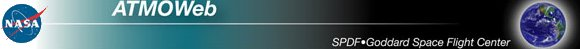SPDF ATMOSPHERIC EXPLORER-D (AE-D) DATA SCATTER PLOT

This interface provides listing and plotting capabilities for the 15-second merged mission data (63 science parameters and 9 orbit parameters) from the AE-D spacecraft. AE-D was in an elliptical orbit (150 - 3800 km) and had an orbit inclination of 90 degrees.

Note: Because of duty cycle limitations there are many data gaps (even several days). The daily ASCII files at our spdf site provide an indication of which days are available.

Time span of the data set: 1975-10-06 - 1976-01-29

### Please Fill the Form below, then Submit Query to start operations:Select an activity
Scatter plot/regression fit (max # points = 30000)     List dataEnter start and stop dates and time
[Format: YYYYMMDDHH, Valid range: 1975100600 - 1976012923]
Start time      Stop timeSelect X and Y variables and optional low/high filter values for any variable
 Variable Name X/Y axes Optional values:Low    High Altitude [km] X-axis Y-axis Latitude [degree] X-axis Y-axis Longitude [degree] X-axis Y-axis Local time [hour] X-axis Y-axis Local Magnetic Time [hour] X-axis Y-axis L-shell parameter [Re] X-axis Y-axis Magnetic inclination [degree] X-axis Y-axis Solar zenith angle [degree] X-axis Y-axis Orbit Number X-axis Y-axis Cylindrical Electrostatic Probe (CEP) Electron temperature [K] X-axis Y-axis Total ion density [cm-3] X-axis Y-axis Spacecraft potential [V] X-axis Y-axis Mean ion mass [AMU] X-axis Y-axis Low-Energy Electron Spectrometer (LEE) Electron total energy flux .2-.8keV [ergs/(cm2 s sr)] X-axis Y-axis Electron total energy flux .8-2keV [ergs/(cm2 s sr)] X-axis Y-axis Electron total energy flux 2-8keV [ergs/(cm2 s sr)] X-axis Y-axis Electron total energy flux 8-30keV [ergs/(cm2 s sr)] X-axis Y-axis Electron average engery [keV] X-axis Y-axis Electron total particle flux .2-30keV [1/(cm2 s sr] X-axis Y-axis Proton total energy flux .2-30keV [ergs/(cm2 s sr] X-axis Y-axis Proton average energy [keV] X-axis Y-axis Proton total particle flux .2-30keV [1/(cm2 s sr] X-axis Y-axis Miniature Electrostatic Accelerometer (MESA) Neutral density [g/cm3] X-axis Y-axis Traverse wind component [m/s] X-axis Y-axis Magnetic Ion Mass Spectrometer (MIMS) H+ [cm--3] X-axis Y-axis D+ [cm-3] X-axis Y-axis He+ [cm-3] X-axis Y-axis O++ [cm-3] X-axis Y-axis N+ [cm-3] X-axis Y-axis O+ [cm-3] X-axis Y-axis Mg+ [cm-3] X-axis Y-axis N2+ [cm-3] X-axis Y-axis NO+ [cm-3] X-axis Y-axis O2+ [cm-3] X-axis Y-axis Neutral Atmosphere Composition Experiment (NACE) N2 [cm-3] X-axis Y-axis O [cm-3] X-axis Y-axis He [cm-3] X-axis Y-axis Ar [cm-3] X-axis Y-axis NO [cm-3] X-axis Y-axis Total Oxygen [cm-3] X-axis Y-axis Neutral Atmosphere Temperature Experiment (NATE) Neutral temperature [K] X-axis Y-axis N2 [cm-3] X-axis Y-axis Total Oxygen (O+2*O2) [cm-3] X-axis Y-axis He [cm-3] X-axis Y-axis Ar [cm-3] X-axis Y-axis UA wind (+ eastward) [m/s] X-axis Y-axis Open-Source Neutral Mass Spectrometer (OSS) N2 [cm-3] X-axis Y-axis O2 [cm-3] X-axis Y-axis He [cm-3] X-axis Y-axis O [cm-3] X-axis Y-axis Ar [cm-3] X-axis Y-axis Special (O/2+O2) [cm-3] X-axis Y-axis N [cm-3] X-axis Y-axis PhotoElectron Spectrometer (PES) Flux 2-3 eV [1/(cm2 eV s sr)] X-axis Y-axis Flux 7-9 eV [1/(cm2 eV s sr)] X-axis Y-axis Flux 12-17 eV [1/(cm2 eV s sr)] X-axis Y-axis Flux 25-30 eV [1/(cm2 eV s sr)] X-axis Y-axis Flux 37-47 eV [1/(cm2 eV s sr)] X-axis Y-axis Flux 100-500 eV [1/(cm2 eV s sr)] X-axis Y-axis Retarding Potential Analyzer (RPA) Ion temperature [K] X-axis Y-axis Total ion density (Ni) [cm-3] X-axis Y-axis Ion drift normal to RPA (VD) [m/s] X-axis Y-axis Spacecraft Potential [V] X-axis Y-axis Transverse vertical ion drift (VD=0) [m/s] X-axis Y-axis Transv. horizontal ion drift (VD=0) [m/s] X-axis Y-axis SIGMA (Delta Ni)/Ni X-axis Y-axis Visible Airglow Experiment CH1: up; CH2: 4278 A [Rayleighs] X-axis Y-axis CH1: down; CH2: 4861 A [Rayleighs] X-axis Y-axis CH1: back; CH2: 5200 A [Rayleighs] X-axis Y-axis CH1: forward; CH2: 5577 A [Rayleighs] X-axis Y-axis CH2: up; CH2: 6300 A [Rayleighs] X-axis Y-axis CH2: down; CH2: 7319 A [Rayleighs] X-axis Y-axisUser may select the fitting method
No fitting      Delta-Y method(N<30000)Advanced plot selections (optional)
 X-axes type: Linear Log10 Y-axes type: Linear Log10 View mode: Create inline GIF output Spawn external GIF viewer PostScript/Landscape PostScript/Portrait Character size (0.5-2.0): Plot symbol: None Plus Asterisk Period Diamond Triangle Square X Symbol size (0.1-4.0): Image size (pixels): X: Y: Connect style: Connect data points Show points onlySend questions about ATMOWeb data to Dr. Dieter Bilitza Send questions about ATMOWeb system/interface to: Dr. Natalia Papitashvili

NASA Official: Robert Candey (Robert.M.Candey@nasa.gov), Head of the Space Physics Data Facility